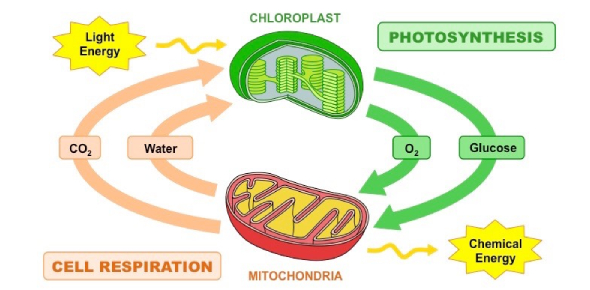# Botany Quiz: Photosynthesis And Cell Respiration

15 Questions | Total Attempts: 6120SettingsCells, just like any living thing, undergo cell respiration to produce energy. This is achieved through photosynthesis, where the plant gets to change the energy from the sunlight to produce glucose and oxygen as byproducts. How well do you understand photosynthesis and cellular respiration? This is a completion grade. You will receive 100 points for Participation for completing this no matter what score you get.

• 1.
Sunlight +6H20 + 6CO2--> C6H1206 +6O2What does the equation above represent?
• A.

Cell Respiration

• B.

Human Respiration

• C.

Photosynthesis

• D.

Solar Energy

• 2.
What is Energy?
• A.

The ability to do work

• B.

The Sun

• C.

The Earth

• D.

Photosynthesis

• 3.
Energy is never created or destroyed, it only is transfered from one thing to another.
• A.

True

• B.

False

• 4.
What are the two reactions in the process of photosynthesis?
• A.

Intermediate Reaction and Beginning reaction

• B.

Light Reactions and the Calvin Cycle

• C.

The Krebs Cycle and Glycolysis

• D.

Respiration and Exhilation

• 5.
What is chemosynthesis?
• A.

The process of making chemicals into chemical energy

• B.

The process of changing the cell

• C.

The process of moving electrons

• D.

The process of linking two things together

• 6.
What are the two types of energy that come from or are used in Photosynthesis and Cell Respiration?
• A.

NDP

• B.

ATP

• C.

• D.

PCP

• 7.
What are the three things that ATP is make of?
• A.

Ribsose Sugar

• B.

Deoxyribose Sugar

• C.

Cell Respiration

• D.

Triphosphate

• E.

Andenine Base

• 8.
Cell Respiration includes three steps.
• A.

True

• B.

False

• 9.
Where does the process of glycolysis take place?
• A.

Cytoplasm

• B.

Nucleus

• C.

Mitochondria

• D.

Chloroplast

• 10.
What step of Cellular Respiration is the majority of the energy produced?
• A.

Calvin Cycle

• B.

Light Reactions

• C.

Krebs Cycle

• D.

Electron Transport Chain

• 11.
C6H1206 +6O2--> 6H20 + 6CO2 + ATPThe reaction shown above is the overall reactions that takes place during which process?
• A.

Photosynthesis

• B.

Cell Respiration

• C.

Human Respiration

• D.

DNA Replication

• 12.
What is a reactant?
• A.

The stuff on the left side of a chemical equation

• B.

The stuff on the right side of a chemical equation

• C.

The stuff on both sides of a chemical equation

• D.

None of the above

• 13.
What is a product?
• A.

The stuff on the left side of a chemical equation

• B.

The stuff on the right side of a chemical equation

• C.

The stuff on both sides of a chemical equation

• D.

None of the above

• 14.
What is an autotroph?
• A.

An animal that eats out of a Trough

• B.

An organism that makes its own food

• C.

An organism that eats using its mouth

• D.

An complex organic compounds

• 15.
Where is the Cell does Photosynthesis happen?
• A.

Nucleus

• B.

Mitochondria

• C.

Chloroplasts

• D.

Cytoplasm

Related TopicsBack to top